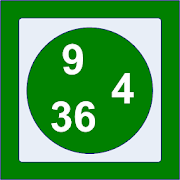Decimal BrainEveryone
Do you need a calculator to work out your shopping bill? Would you like to get some practice in doing decimal arithmetic in your head? This app, "Decimal Brain", is a trainer to help you do decimal arithmetic in your head, which randomly generates sets of 10 questions for each level. There are controls to select specific question sets, move to the next question, and view the current questions and answers and best scores for every level played in the current session.

The levels included are:
• 1 : + => 10 questions about the addition of 2 decimal numbers between 0.2 and 1.1.
• 2 : - => 10 questions about the subtraction of 2 decimal numbers between 0.2 and 1.8.
• 3 : x => 10 questions about the multiplication of 2 decimal numbers between 0.2 and 1.0.
• 4 : x ! => 10 questions about the multiplication of 2 decimal numbers between 0.6 and 1.2.
• 5 : (+)- => 10 questions about the addition and subtraction of 3 decimal numbers between 0.2 and 1.8.
• 6 : (x)+ => 10 questions about the multiplication and addition of 3 decimal numbers between 0.2 and 1.0.
• 7 : (-)x => 10 questions about the substraction and multiplication of 3 decimal numbers between 0.2 and 1.2.
• 8 : (+)x => 10 questions about the addition and multiplication of 3 decimal numbers between 0.2 and 1.0.
Collapse

Review Policy

What's New

1.0.0 New release.
Collapse

Eligible for Family LibraryEligible if bought after 7/2/2016. Learn More
Updated
January 22, 2019
Size
263k
Installs
0+
Current Version
1.0.0
Requires Android
2.3.3 and up
Content Rating
Everyone
Permissions
Offered By
Holland Numerics
Developer
94 Green Drift Royston Hertfordshire SG8 5BT United Kingdom